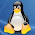## Friday, 26 June 2009

### Broken axis revisited

In one of my first posts, I described a method by which one can draw broken axes in gnuplot. Now, that method suffers from many problems, most notably that it relied on gnuplot 4.3 (still in development), and one had to do almost everything by hand. Today we will look at a different method, which eliminates these problems, and which requires very little human intervention. So, we would like to have something like this graph:

(This is just a sin function, broken at 4.5, and for the right hand side, displaced by 3). The recipe that we are going to follow takes only a couple of lines. But, let us see the code!
```reset
A=4.5
B=3.0
C=0
D=10
E=1
eps=0.05*E
eps2=0.005*(D-C)
unset key
f(x) = (x<A?1:1/0)
g(x) = (x>A?1:1/0)
h(x) = (x<A?x:x+B)
set xtics 0, 2, A
set xtics add (gprintf("%.0f", 6+B) 6)
set xtics add (gprintf("%.0f", 8+B) 8)
set xtics add (gprintf("%.0f", 10+B) 10)

set xlabel 'Time [s]'
set ylabel 'Position [m]'
set yrange [-E:E]
set arrow 1 from A-eps2, -E to A+eps2, -E nohead lc rgb "#ffffff" front
set arrow 2 from A-eps2, E to A+eps2, E nohead lc rgb "#ffffff" front
set arrow 3 from A-eps-eps2, -E-eps to A+eps-eps2, -E+eps nohead front
set arrow 4 from A-eps+eps2, -E-eps to A+eps+eps2, -E+eps nohead front
set arrow 5 from A-eps-eps2, E-eps to A+eps-eps2, E+eps nohead front
set arrow 6 from A-eps+eps2, E-eps to A+eps+eps2, E+eps nohead front
plot [C:D] f(x)*sin(x) w l lt 1, g(x)*sin(h(x)) w l lt 1```

The first several lines are just definitions: 'A' is the position at which we want to break the line, 'B' is the value by which the right hand side of the graph will be shifted, 'C', 'D' and 'E' are just definitions for the x and y ranges, while 'eps' and 'eps2' will be needed for the drawing of the slanted tics representing the discontinuity in the axes.

The first really important definition is that of f(x), g(x) and h(x), which are helper functions (green lines). In all three cases, we use the ternary operator that I discussed in my previous post. Basically, f(x) returns 1, if x is smaller than 'A', g(x) does just the opposite, while h(x) produces a shift of its argument, if x is larger than 'A'.

Then we set the labels on the x axis (blue line in the code). But watch out: we do it only up to 'A', because the axis is going to be broken at 'A'. In order to make up for the missing tic marks, we add them in the next three lines (red). We can save some work, and can eliminate the possibility of messing something up, if we ask gnuplot to compute the values for us; this is done by the string conversion function gprintf, and adding the converted string.
`set xtics add (gprintf("%.0f", 10+B) 10)`

The last bit is drawing the break points on the axis. In order to do so, we draw 6 arrows with no heads. Note that the first two arrows are white, and they serve as a beauty plaster: we put them on top of the axes, to give the impression that the axes are broken. Also note that since arrows are drawn earlier than axes and borders, we have got to explicitly instruct gnuplot to bring the arrows to the front of the figure. The last four line segments are slightly slanted, to give a more appealing look to the graph. Once we are done with the set up of the figure, we can plot the function. The only thing we have to keep in mind is that the right hand side should be shifted by 'B', which is done by calling h(x) in the argument of sin(x).

The procedure for the y axis is very similar, so I just dodge the discussion of that. I should point out that you can easily change the angle of the last 4 arrows by multiplying the shift of the y coordinates of the end points by something, and it is just as easy to move them farther from each other, if you replace the definition of 'eps2'. Modifying 'eps' will change their length, but not their angle.

Till next time!

1.This is awesome. I can't seem to get my titles to show up, though.

plot data using 1:2 with lines title 'Set 1'

Produces the lines but not the typical legend in the upper right. Do you have a suggestion for this?

2.Figured it out. Removing "unset key" did it. I'm a novice and didn't realize what that line did... now it makes complete sense that this would have... duh... removed the key!

3.Is it possible to do curve fitting in Gnuplot with constraint on the fitting parameters??

Thanks

1.Not directly. The fitting parameter should be set as a function that is itself constrained. For example: if you need to constrain the parameter between 0 and 1 you could use a parameter within (sin())^2. This may be a bit of a problem in large data sets (many iterations) and many times results in divergence requiring time consuming fine tuning.

4.Yes. I discuss this problem in
http://gnuplot-tricks.blogspot.com/2009/12/restricting-fit-parameters.html

I hope this help,
Zoltán